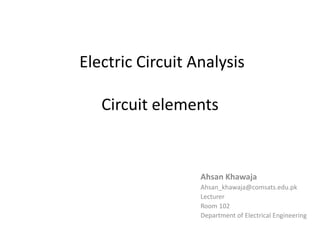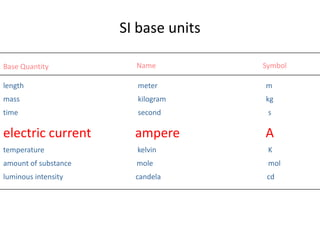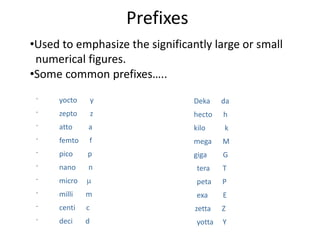Successfully reported this slideshow.

# Electrical Circuit - Lecture#2×

1 of 32
1 of 32

# Electrical Circuit - Lecture#2

## More Related Content

### Electrical Circuit - Lecture#2

1. 1. Electric Circuit Analysis Circuit elements Ahsan Khawaja Ahsan_khawaja@comsats.edu.pk Lecturer Room 102 Department of Electrical Engineering
2. 2. Base Quantity SI base units Name Symbol length meter m mass kilogram kg time second s electric current ampere A temperature kelvin K amount of substance mole mol luminous intensity candela cd
3. 3. Prefixes •Used to emphasize the significantly large or small numerical figures. •Some common prefixes….. - yocto y - zepto z - atto a - femto f - pico p - nano n - micro - milli m - centi c - deci d Deka da hecto h kilo k mega M giga G tera T peta P exa E zetta Z yotta Y
4. 4. Charge • In SI system, charge is measured in coulombs (C). • The charge on an electron is -1.602 x 10-19 C. • Hence 1 C of charge, there are 1/(1.602 x 10-19) = 6.24 x 1018 electrons. • Denoted by ‘ q ’.
5. 5. Current • ‘I’, is the rate at which charge is transferred or "flows" through a device. • Mathematically, dq(t) dt i(t) = where i = the current in amperes (A) q = the charge in coulombs (C) the amount of charge passing through a device per unit of time. ampere is coulomb per second.
6. 6. i t 0 5 10 15 20 -1 -0.5 0 0.5 1 0 5 10 15 20 -0.8 -0.6 -0.4 -0.2 0 0.2 0.4 0.6 0.8 1 0 5 10 15 20 -3 -2 -1 0 1 2 3 4 5 0 5 10 15 20 0 0.2 0.4 0.6 0.8 1 Direct Current (dc) Alternating/Sinusoidal Current (ac) Exponential Current Damped Sinusoidal Current Transient Current Types of current
7. 7. Current sign convention Proper definition of current vector requires: •direction of the arrow(polarity). •value (magnitude) A negative current of - A flowing in one direction is the same as a current of + A flowing in the opposite direction.
8. 8. Electronic VS conventional current Electrons flow from negative terminal to positive terminal but conventional current flows in the opposite direction.
9. 9. Example DC current. Find the charge passing through a point in a conductor if the current passing through the conductor has the waveform indicated. i t 2 A Solution: The current does not change with time. Hence, a charge of 2 C is passing through the point each second.
10. 10. Example For the given current, which is given in graphical form, find the charge passing through a given point in the time interval 0-4 s. t (sec) 10 A 10 2 43 i(t)
11. 11. t (sec) 10 A 10 2 43 i(t) Solution: 4 0 q(t) i(t)dt 1 2 0 1 10tdt 10dt 3 4 2 3 ( 10t 30)dt (10t 30)dt Ans = + - + C = C
12. 12. Voltage • Recognized by the 18th century Italian physicist Allessandro Antonio Volta. • Voltage" or "potential difference” is defined as the energy required to move a unit charge through an element. • Measured in volts (V). • Mathematically, dw dq V w = the energy in joules (J volt is joule per coulomb.
13. 13. Voltage sign convention
14. 14. DC and AC voltage • Like electric current, a constant voltage is called a “dc voltage” and is represented by V”, whereas a sinusoidally time-varying voltage is called an “ac voltage” and is represented by “v • A dc voltage is usually produced by a battery and an ac voltage is produced by an electric generator.
15. 15. Passive Sign Convention • The passive sign convention is satisfied when the current enters through the positive(plus- marked) terminal of an element as shown. circuit elementv – + i i . . . The passive sign convention.
16. 16. Example Solution: p = W p = W p = W Total power W W + W 600 W + 300 V – + 60 V – + 60 V – + 240 V – 2 A 2 A 1.5 A 0.5 A 3 4 2 Find the power dissipated for the following circuit. Hint: P = V x I What is the power generated by the source?
17. 17. Voltage VS Current The voltage across an element can be • linearly proportional to the current through it (a resistor) • proportional to derivative (an inductor) of the current with respect to time. • proportional to integral (a capacitor) of the current with respect to time.
18. 18. Voltage and Current Sources Two types of basic electrical elements: • passive elements.(dissipate power) • active elements. (generate power) Active elements such as voltage and current sources can be classified as either independent or dependent sources.
19. 19. Independent Voltage Sources An ideal independent source is an active element that can supply a specified voltage or current that is completely independent of a current or voltage elsewhere in the circuit. vsVs (a) and (b) DC independent voltage source symbols. (c) AC independent voltage source symbol. (a) (b) (c) V
20. 20. Independent Current Sources (a) (b) (a) A time-varying independent current source. (b) A constant (DC) independent current source. i I
21. 21. Dependent Sources • Dependent, or controlled, source is a source in which the source voltage or current is determined by another voltage or current elsewhere in the circuit being analyzed. • It is usually represented by diamond-shaped symbol. (a) (b) (a) A dependent voltage source. (b) A dependent current source. v i
22. 22. Dependent sources of four types 1. A voltage-controlled voltage source (VCVS) 2. A current-controlled voltage source (CCVS). 3. A voltage-controlled current source (VCCS). 4. A current-controlled current source (CCCS).
23. 23. VCVS i v = vx v = vx vx is somewhere (not shown) and is a constant. i = whatever
24. 24. CCVS i v = ix v = ix i = whatever ix is somewhere (not shown) and is a constant.
25. 25. VCCS i = vx i = vx vx is somewhere (not shown) and is a constant. v = whateverv
26. 26. CCCS i = ix i = ix v = whatever ix is somewhere (not shown) and is a constant. v
27. 27. Resistance ‘R’ • Unit of resistance is ohms (Ω) 1 Ω = 1 V / A • Resistance in a circuit arises due to collisions between the electrons carrying the current with the fixed atoms inside the conductor. • Experiments show that for many materials, including most metals, the resistance remains constant over a wide range of applied voltages or currents.
28. 28. Ohm’s Law • Ohm’s Law is an empirical relationship that is valid only for certain materials – Materials that obey Ohm’s Law are said to be ohmic V V = R I Ohm’s law
29. 29. Parallel connection Series connection R1 R2 R3 R1 R2 R3 Req = R1 + R2 + R3 1/Req = 1/R1+1/R2+1/R3
30. 30. Q2. What is the ratio of the current flowing through each resistor (I1:I2) in the circuit? 1. 1:1 2. 3:1 3. 1:4 4. Need more info. 6 V R1 = 10 R2 = 30
31. 31. Ra Rb 1/Req = 1/Ra + 1/Rb Req is smaller than Ra and Rb 20 25 Req ≈ 10 1000 = 1k 2 Req < 2 Practically all the current flows Though the bottom one!! Practical considerations for ‘R’